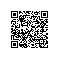# 正则表达式（java）

• ^[0-9][1-9][0-9]$//匹配正整数 • ^((-\d+)|(0+))$　　//匹配非正整数（负整数 + 0）

• ^-[0-9][1-9][0-9]$//匹配负整数 • ^-?\d+$　　　　//匹配整数

• ^\d+(.\d+)?$//匹配非负浮点数（正浮点数 + 0） • ^(([0-9]+.[0-9][1-9][0-9])|([0-9][1-9][0-9].[0-9]+)|([0-9][1-9][0-9]))$　　//匹配正浮点数

• ^((-\d+(.\d+)?)|(0+(.0+)?))$//匹配非正浮点数（负浮点数 + 0） • ^(-(([0-9]+.[0-9][1-9][0-9])|([0-9][1-9][0-9].[0-9]+)|([0-9][1-9][0-9])))$　　//匹配负浮点数

• ^(-?\d+)(.\d+)?$//匹配浮点数 • ^[A-Za-z]+$　　//匹配由26个英文字母组成的字符串

• ^[A-Z]+$//匹配由26个英文字母的大写组成的字符串 • ^[a-z]+$　　//匹配由26个英文字母的小写组成的字符串

• ^[A-Za-z0-9]+$//匹配由数字和26个英文字母组成的字符串 • ^\w+$　　//匹配由数字、26个英文字母或者下划线组成的字符串

• ^[\w-]+(.[\w-]+)*@[\w-]+(.[\w-]+)+$//匹配email地址 • 匹配中文字符的正则表达式： [\u4e00-\u9fa5] • 匹配双字节字符(包括汉字在内)：[^\x00-\xff] • 匹配空行的正则表达式：\n[\s| ]*\r • 匹配HTML标记的正则表达式：/<(.)>.</\1>|<(.*) />/ • 匹配首尾空格的正则表达式：(^\s)|(\s$)

• 正则表达式用例
• 1、^\S+[a-z A-Z]$不能为空 不能有空格 只能是英文字母 • 2、\S{6,} 不能为空 六位以上 • 3、^\d+$ 不能有空格 只能是数字
• 4、(.*)(.jpg|.bmp)$只能是jpg和bmp格式 • 5、^\d{4}-\d{1,2}-\d{1,2}$ 只能是2004-10-22格式
• 6、^0$至少选一项 • 7、^0{2,}$ 至少选两项
• 8、^[\s|\S]{20,}$不能为空 二十字以上 • 9、^+?a-z0-9*@([a-z0-9]+(.|-))+[a-z]{2,6}$邮件
• 10、\w+([-+.]\w+)@\w+([-.]\w+).\w+([-.]\w+)([,;]\s\w+([-+.]\w+)@\w+([-.]\w+).\w+([-.]\w+)) 输入多个地址用逗号或空格分隔邮件
• 11、^(([0-9]+))?[0-9]{7,8}$电话号码7位或8位或前面有区号例如（022）87341628 • 12、^[a-z A-Z 0-9 *]+@[a-z A-Z 0-9 *]+(.[a-z A-Z 0-9 *]+)+(,[a-z A-Z 0-9 *]+@[a-z A-Z 0-9 *]+(.[a-z A-Z 0-9 ]+)+)$
• 只能是字母、数字、下划线；必须有@和.同时格式要规范 邮件
• 13 ^\w+@\w+(.\w+)+(,\w+@\w+(.\w+)+)*$上面表达式也可以写成这样子，更精练。 • 14 ^\w+((-\w+)|(.\w+))@\w+((.|-)\w+).\w+$ [/size]使用钉钉扫一扫加入圈子
+ 订阅